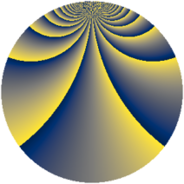# Properties

 Label 2736.2.fxLevel $2736$ Weight $2$ Character orbit 2736.fx Rep. character $\chi_{2736}(127,\cdot)$ Character field $\Q(\zeta_{18})$ Dimension $300$ Sturm bound $960$

# Related objects

## Defining parameters

 Level: $$N$$ $$=$$ $$2736 = 2^{4} \cdot 3^{2} \cdot 19$$ Weight: $$k$$ $$=$$ $$2$$ Character orbit: $$[\chi]$$ $$=$$ 2736.fx (of order $$18$$ and degree $$6$$) Character conductor: $$\operatorname{cond}(\chi)$$ $$=$$ $$76$$ Character field: $$\Q(\zeta_{18})$$ Sturm bound: $$960$$

## Dimensions

The following table gives the dimensions of various subspaces of $$M_{2}(2736, [\chi])$$.

Total New Old
Modular forms 3024 300 2724
Cusp forms 2736 300 2436
Eisenstein series 288 0 288

## Trace form

 $$300q + O(q^{10})$$ $$300q + 12q^{13} - 18q^{41} + 150q^{49} + 36q^{53} + 60q^{61} - 108q^{65} - 120q^{73} - 72q^{77} + 72q^{85} - 36q^{89} - 18q^{97} + O(q^{100})$$

## Decomposition of $$S_{2}^{\mathrm{new}}(2736, [\chi])$$ into newform subspaces

The newforms in this space have not yet been added to the LMFDB.

## Decomposition of $$S_{2}^{\mathrm{old}}(2736, [\chi])$$ into lower level spaces

$$S_{2}^{\mathrm{old}}(2736, [\chi]) \cong$$ $$S_{2}^{\mathrm{new}}(76, [\chi])$$$$^{\oplus 9}$$$$\oplus$$$$S_{2}^{\mathrm{new}}(228, [\chi])$$$$^{\oplus 6}$$$$\oplus$$$$S_{2}^{\mathrm{new}}(304, [\chi])$$$$^{\oplus 3}$$$$\oplus$$$$S_{2}^{\mathrm{new}}(684, [\chi])$$$$^{\oplus 3}$$$$\oplus$$$$S_{2}^{\mathrm{new}}(912, [\chi])$$$$^{\oplus 2}$$